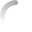# Centripetal Taxation: The MathIf you are not interested in the mathematical underpinnings, you can skip this explanation and see Centripetal Taxation in action.

This Centripetal Taxation system is based on an integral of a sigmoid function. In a perhaps too-elliptical paper I wrote a while back I originally chose the Logistic Function as the sigmoid, mostly because its range is naturally in the unit interval, $[0,1]$. I now use a simpler sigmoid function with a range in the unit interval that does not require (possibly high) powers of $e$.

Here is the sigmoid function:
whose integral is:
We now need to select a value for the following:
and choose a value for $C$ such that $A(z)=0$:
We then set the following:
and define this:
Now we're ready to calculate a tax: